# Fluid Dynamics¶

Important: Please read the installation page for details about how to install the toolboxes. $\newcommand{\dotp}{\langle #1, #2 \rangle}$ $\newcommand{\enscond}{\lbrace #1, #2 \rbrace}$ $\newcommand{\pd}{ \frac{ \partial #1}{\partial #2} }$ $\newcommand{\umin}{\underset{#1}{\min}\;}$ $\newcommand{\umax}{\underset{#1}{\max}\;}$ $\newcommand{\umin}{\underset{#1}{\min}\;}$ $\newcommand{\uargmin}{\underset{#1}{argmin}\;}$ $\newcommand{\norm}{\|#1\|}$ $\newcommand{\abs}{\left|#1\right|}$ $\newcommand{\choice}{ \left\{ \begin{array}{l} #1 \end{array} \right. }$ $\newcommand{\pa}{\left(#1\right)}$ $\newcommand{\diag}{{diag}\left( #1 \right)}$ $\newcommand{\qandq}{\quad\text{and}\quad}$ $\newcommand{\qwhereq}{\quad\text{where}\quad}$ $\newcommand{\qifq}{ \quad \text{if} \quad }$ $\newcommand{\qarrq}{ \quad \Longrightarrow \quad }$ $\newcommand{\ZZ}{\mathbb{Z}}$ $\newcommand{\CC}{\mathbb{C}}$ $\newcommand{\RR}{\mathbb{R}}$ $\newcommand{\EE}{\mathbb{E}}$ $\newcommand{\Zz}{\mathcal{Z}}$ $\newcommand{\Ww}{\mathcal{W}}$ $\newcommand{\Vv}{\mathcal{V}}$ $\newcommand{\Nn}{\mathcal{N}}$ $\newcommand{\NN}{\mathcal{N}}$ $\newcommand{\Hh}{\mathcal{H}}$ $\newcommand{\Bb}{\mathcal{B}}$ $\newcommand{\Ee}{\mathcal{E}}$ $\newcommand{\Cc}{\mathcal{C}}$ $\newcommand{\Gg}{\mathcal{G}}$ $\newcommand{\Ss}{\mathcal{S}}$ $\newcommand{\Pp}{\mathcal{P}}$ $\newcommand{\Ff}{\mathcal{F}}$ $\newcommand{\Xx}{\mathcal{X}}$ $\newcommand{\Mm}{\mathcal{M}}$ $\newcommand{\Ii}{\mathcal{I}}$ $\newcommand{\Dd}{\mathcal{D}}$ $\newcommand{\Ll}{\mathcal{L}}$ $\newcommand{\Tt}{\mathcal{T}}$ $\newcommand{\si}{\sigma}$ $\newcommand{\al}{\alpha}$ $\newcommand{\la}{\lambda}$ $\newcommand{\ga}{\gamma}$ $\newcommand{\Ga}{\Gamma}$ $\newcommand{\La}{\Lambda}$ $\newcommand{\si}{\sigma}$ $\newcommand{\Si}{\Sigma}$ $\newcommand{\be}{\beta}$ $\newcommand{\de}{\delta}$ $\newcommand{\De}{\Delta}$ $\newcommand{\phi}{\varphi}$ $\newcommand{\th}{\theta}$ $\newcommand{\om}{\omega}$ $\newcommand{\Om}{\Omega}$

This numerical tour explores fluid dynamics for image generation.

In :
using PyPlot
using NtToolBox
using Interpolations


## Velocity Flow Field¶

A velocity flow is simply a 2-D vector field $V = (V_i)_{i=1}^N \in \RR^{n \times n \times 2}$ where $V_i \in \RR^2$ is one of the $N=n \times n$ vectors at a position indexed by $i$.

It can be generated as a realization of Gaussian process. The blurring creates correlations in the flow.

In :
n = 128
V = perform_blurring(randn(n,n,2),, "per");


Subsampling display operator.

In :
myplot = V-> plot_vf(V[1:6:n,1:6:n, :])

Out:
(::#1) (generic function with 1 method)

We can display the vector field using arrow.

In :
figure(figsize = (7,7))
myplot(V)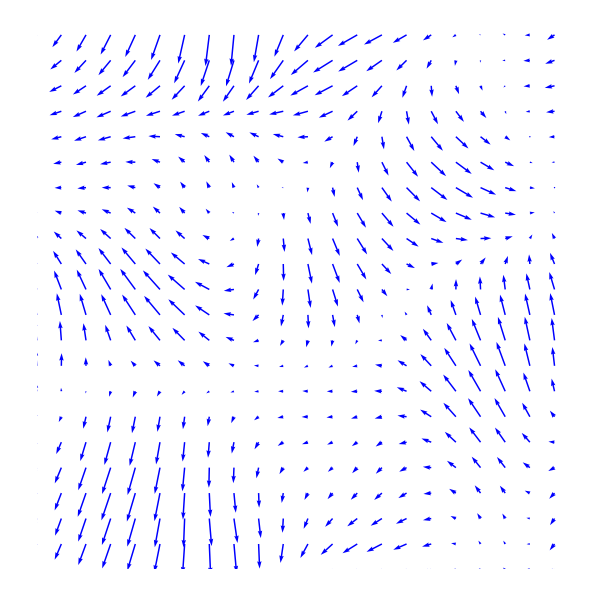We can renormalize the flow, which enhances the singularities. It defines $\tilde V$ as $\tilde V_i = V_i/\norm{V_i}$.

In :
Normalize = V -> V ./ repeat( max(1e-9,sqrt.(sum(V.^2, 3))) , outer=(1,1,2));


Display.

In :
figure(figsize = (7,7))
myplot(Normalize(V))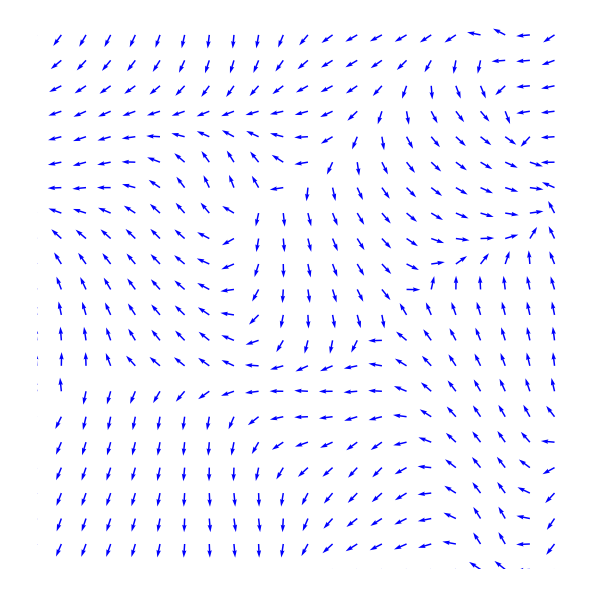## Incompressible Flows¶

An incompressible flow has a vanishing divergence. The set of vector incompressible flow defines a sub-space of $\RR^{n \times n \times 2}$ $$\Ii = \enscond{V}{ \text{div}(V)=0 } \qwhereq \text{div}(V) = \pd{V}{x_1} + \pd{V}{x_2} \in \RR^{n \times n}.$$ Here $\pd{}{x_s}$ for $s=1,2$ are finite differences approximation of the horizontal and vertical derivative operators (we suppose here periodic boundary conditions).

The orthogonal projection $U = \text{Proj}_{\Ii}(V)$ on $\Ii$ is computed by solving a Poisson equation $$U = V-\nabla A \qwhereq \Delta A = \text{div}(V).$$

This is especially simple for periodic boundary conditions since $A$ can be computed over the Fourier domain as $$\forall \om \neq 0, \quad \hat A(\om) = \frac{\hat Y(\om)}{\mu(\om)} \qwhereq Y = \text{div}(V) \qandq \mu(\om_1,\om_2) = -4 \sin(\om_1 \pi / n)^2 -4 \sin(\om_2 \pi / n)^2$$ and $\hat A(0)=0$.

Compute the kernel $\mu(\om)$.

In :
Y, X = meshgrid(0:n-1,0:n-1)
mu = sin.(X*pi/n).^2
mu = -4*(mu + mu')
mu[1,1] = 1;


Computation of $A$.

In :
A = V -> real.(ifft(fft(Div(V[:,:,1], V[:,:,2], "per"))./mu))

Out:
(::#5) (generic function with 1 method)

Projection on incompressible flows.

In :
ProjI = V -> V - Grad(A(V), "per")

Out:
(::#7) (generic function with 1 method)

Display $U=\text{Proj}_{\Ii}(V)$.

In :
U = ProjI(V)

figure(figsize=(7,7))
myplot(U)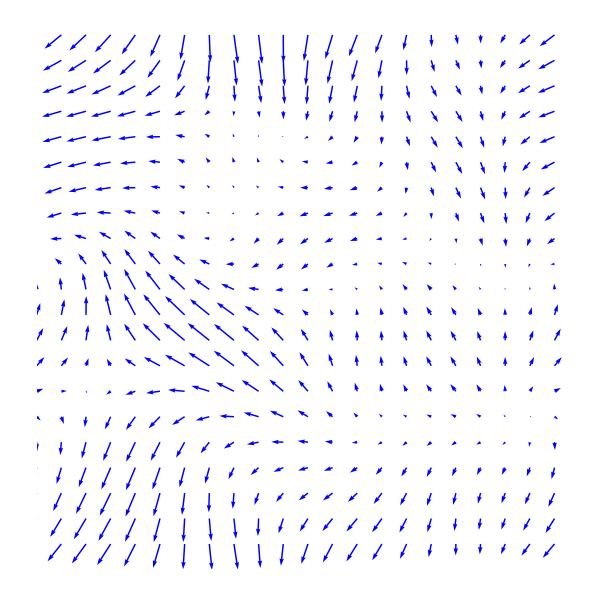Display $W=U-V$ the irrotational component of $V$.

In :
figure(figsize=(7,7))
myplot(V-U)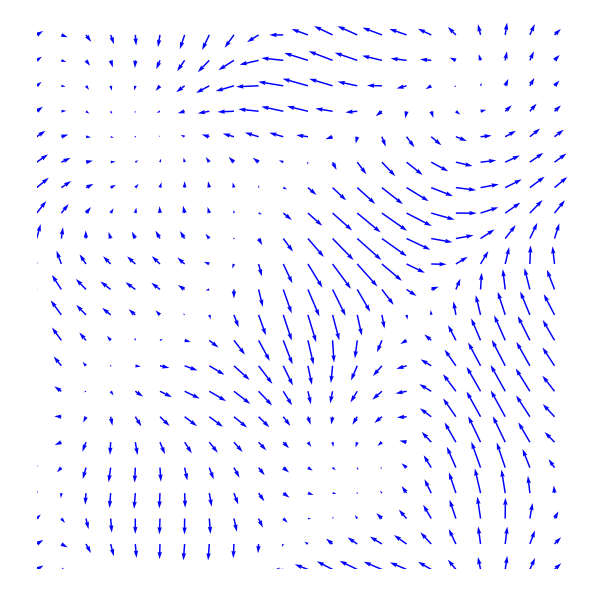Note that the decomposition $V=U+W$ is called the Hoge decomposition of the vector field.

## Image Advection Along the Flow¶

A flow defines a warping operator that transport the content of an image along the streaming of the flow.

We load an image $f$.

In :
f = load_image("NtToolBox/src/data/lena.png", 2*n)
f = f[n-Base.div(n,2):n+Base.div(n,2)-1, n-Base.div(n,2):n+Base.div(n,2)-1];


Given some vector field $U$, the warping operator $f_1 = \Ww_U(f)$ along the flow is defined $$f_1(x) = f(x+U(x))$$ i.e. it advects the values of $f$ by the vector field $U$ to obtain the values of $f_1$.

We define $U$ as a scaled normalized incompressible flow.

In :
U = Normalize(ProjI(V));


Helper function: enforce periodicity.

In :
periodic = P -> cat(3, mod(P[:,:,1]-1,n)+1, mod(P[:,:,2]-1,n)+1 );


Helper function: extend an image by 1 pixel to avoid boundary problems.

In :
extend1 = f -> [f f[:,1]]
extend = f -> extend1(extend1(f)')';


Helper function: bilinear interpolation on a grid.

In :
f1 = extend(f)
itp = interpolate((1:size(f1,1), 1:size(f1,2)), f1, Gridded(Linear()))
size(itp)

Out:
(129,129)
In :
function myinterp(P1,f1,Pi)
itp = interpolate((1:size(f1,1), 1:size(f1,2)), f1, Gridded(Linear()))
itpd = zeros(size(Pi)[1:2])
for i in 1:size(Pi,1)
for j in 1:size(Pi,2)
itpd[i,j] = itp[Pi[i,j,1], Pi[i,j,2]]
end
end
return itpd
end;


First we compute the initial and wraped grids.

In :
(Y,X) = meshgrid(1:n,1:n)
P = cat(3, X,Y)
(Y1,X1) = meshgrid(1:n+1,1:n+1)
P1 = cat(3, X1,Y1);


Defines the warping operator $\Ww_U$.

In :
W =  (f, U) -> myinterp(P1, extend(f), periodic(P - U));


Display a warped image $\Ww_{\rho U}(f)$ for some scaling $\rho$.

In :
rho = 2
figure(figsize = (5,5))
imageplot(W(f, rho*U))Exercise 1

Display $\Ww_{\rho U}(f)$ for various values of $\rho$.

In :
include("NtSolutions/graphics_5_fluids/exo1.jl")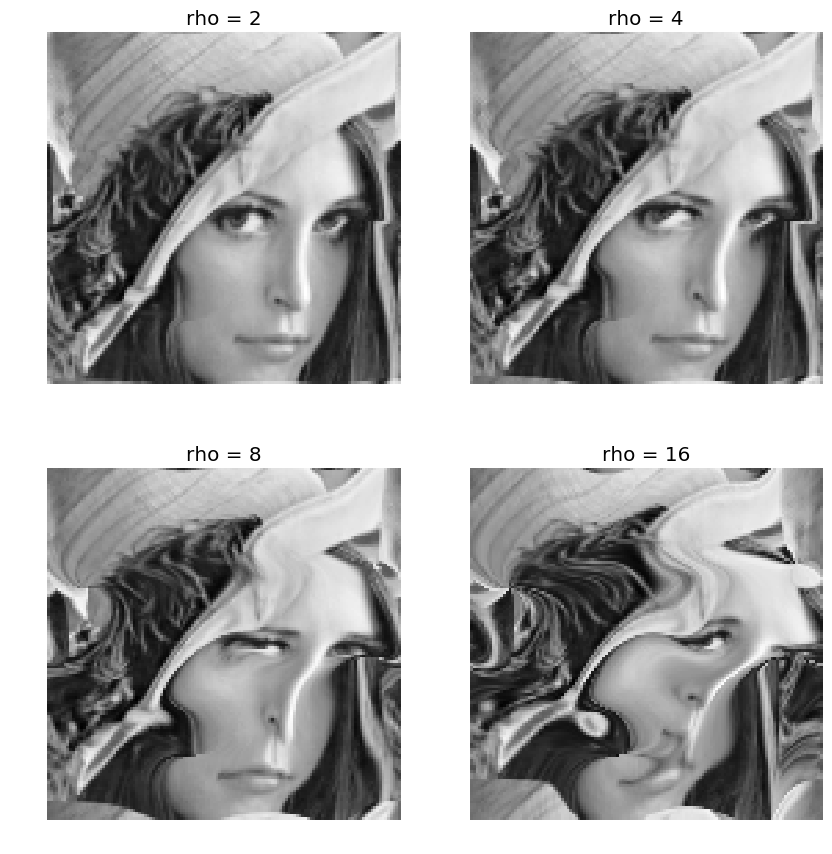In :
## Insert your code here.


Exercise 2

Define an iterative scheme via: $$f^{(\ell+1)} = \Ww_{\rho U}(f^{(\ell)}).$$ Display the result $f^{(\ell)}$, which corresponds approximately to solving an advection equation at time $t=\ell \rho$.

In :
include("NtSolutions/graphics_5_fluids/exo2.jl")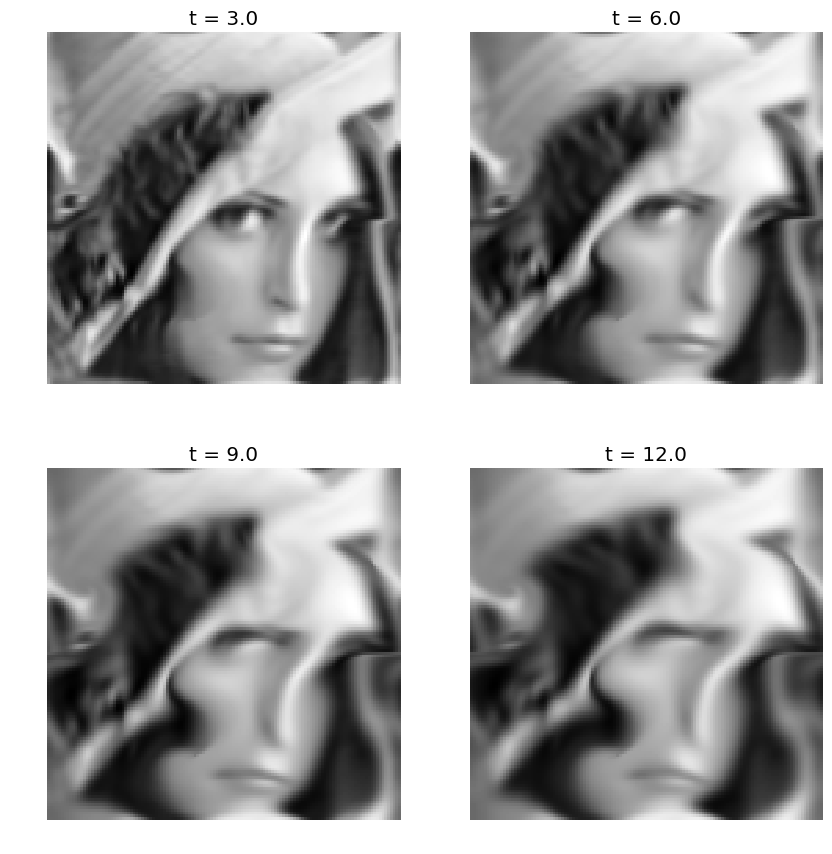In :
## Insert your code here.


## Fluid Dynamics¶

Fluid dynamics solves the incompressible Navier-Stokes equations to evolve in time the vector field.

We discribe here a simple algorithm introduced in:

J. Stam, Stable Fluids, SIGGRAPH'99, 1999, p. 121-128.

It proposes a semi-implicit scheme for the resolution of the Navier Stockes equations for the movement of incompressible fluids $$\pd{V}{t} = \text{Proj}_{\Ii}\pa{ -(V \cdot \nabla) V + \nu \Delta V + W }.$$ Here $\nu \geq 0$ is the viscosity of the fluid, $W$ is a source term, $\Delta$ is the Laplacian, and $-(V \cdot \nabla) V$ is the non-linear self-advection, where we have used the short-hand notation $V \cdot \nabla$ for the derivative operator along a flow $V$: $$(V \cdot \nabla)U = ( V_1 \pd{U_1}{x_1} + V_2 \pd{U_1}{x_2}, V_1 \pd{U_2}{x_1} + V_2 \pd{U_2}{x_2} ).$$

In order to visualize the flow, we also advect and diffuse along the flow a density $g$ of particules, which is a scalar field. Once $V$ has been computed, it follows a linear PDE $$\pd{g}{t} = -(V \cdot \nabla) g + \mu \Delta g + h$$ with some initial condition at time $t=0$, where $h$ is a source for the density.

In practice, we solve this PDE in parallel to the PDE for $V$.

In the following, we use $W=0$ and $h=0$ (no sources).

Set the viscosity $\nu$ for the velocity field.

In :
nu = 1/10

Out:
0.1

We use a larger viscosity $\mu$ for the evolution of the density of particules.

In :
mu = 2*nu

Out:
0.2

Extend the warping operator $\Ww_U$ to work with vector fields as input. This will apply $\Ww_U$ on each channel of the vector field (X and Y coordinates).

In :
Wt = (V,U) -> cat(3, W(V[:,:,1],U), W(V[:,:,2],U) );


We discretize the PDE's using some time step $\tau$.

In :
tau = .5;


The algorithm computes $V^{(\ell)}$ at iteration $\ell$ which is an approximation of the PDE solution at time $\ell \tau$. It is computed itertatively as $$\tilde V^{(\ell)} = \Ww_{\tau V^{(\ell)}}( V^{(\ell)} ) \qandq V^{(\ell+1)} = \text{Proj}_{\Ii}\pa{ \tilde V^{(\ell)} + \tau\nu\Delta \tilde V^{(\ell)} + \tau W }$$

It computes in parallel the evolution of the density as $$\tilde g^{(\ell)} = \Ww_{\tau V^{(\ell)}}( g^{(\ell)} ) \qandq g^{(\ell+1)} = \tilde g^{(\ell)} + \tau\nu\Delta \tilde g^{(\ell)} + \tau h$$

Set the initial field $V=V^{(0)}$ at time $t=0$.

In :
V = Normalize(ProjI(V));


Set the initial density $g=g^{(0)}$ at time $t=0$.

In :
g = copy(f);


The first step is to advect the vector field $V$ and $g$ along the flow $V$ itself. This corresponds to an implict discretization of the term $-(V \cdot \nabla) V$.

In :
g = W(g, tau*U)
V = Wt(V, tau*U);


We implement the Laplacian using finite difference.

In :
s1 = [2:n; 1]; s2 = [n; 1:n-1];

function Delta(g)
delta = 1/4.*(g[s1,:,:] + g[s2,:,:] + g[:,s1,:] + g[:,s2,:]) - g
if size(delta,3)==1
delta = delta[:,:,1]
end
return delta
end;


The second step is to diffuse the vector field and the density.

In :
V = V + tau*nu*Delta(V)
g = g + tau*mu*Delta(g);


The last step is to ensure incompressibility of $V$ by projecting on $\Ii$.

In :
V = ProjI(V);


Exercise 3

Compute the fluid dynamic by iterating these steps.

In :
include("NtSolutions/graphics_5_fluids/exo3.jl")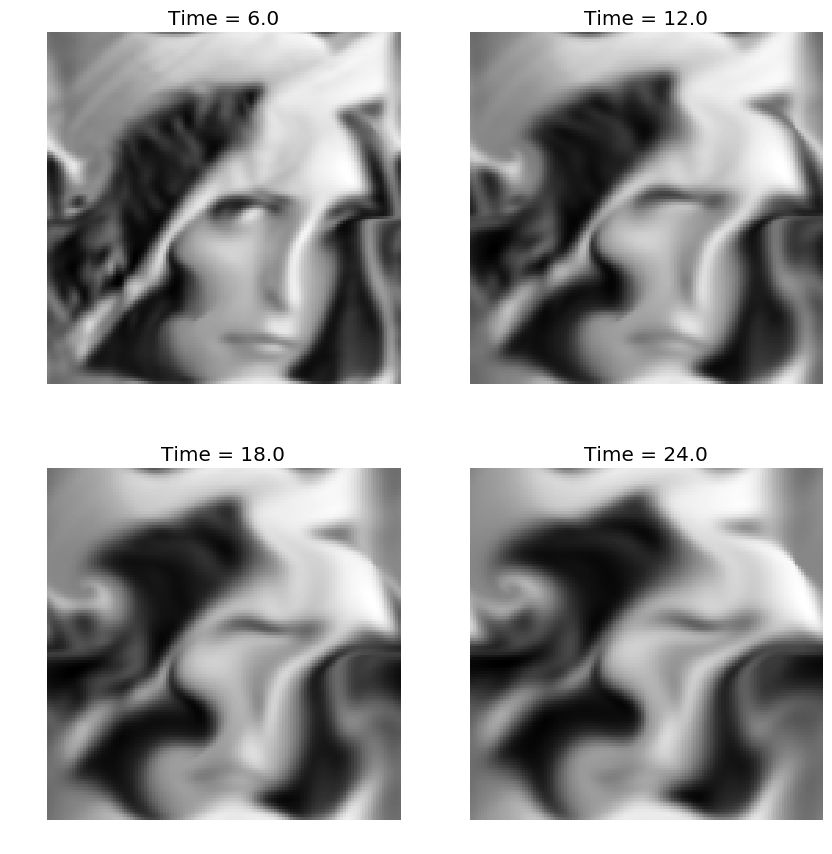In :
## Insert your code here.